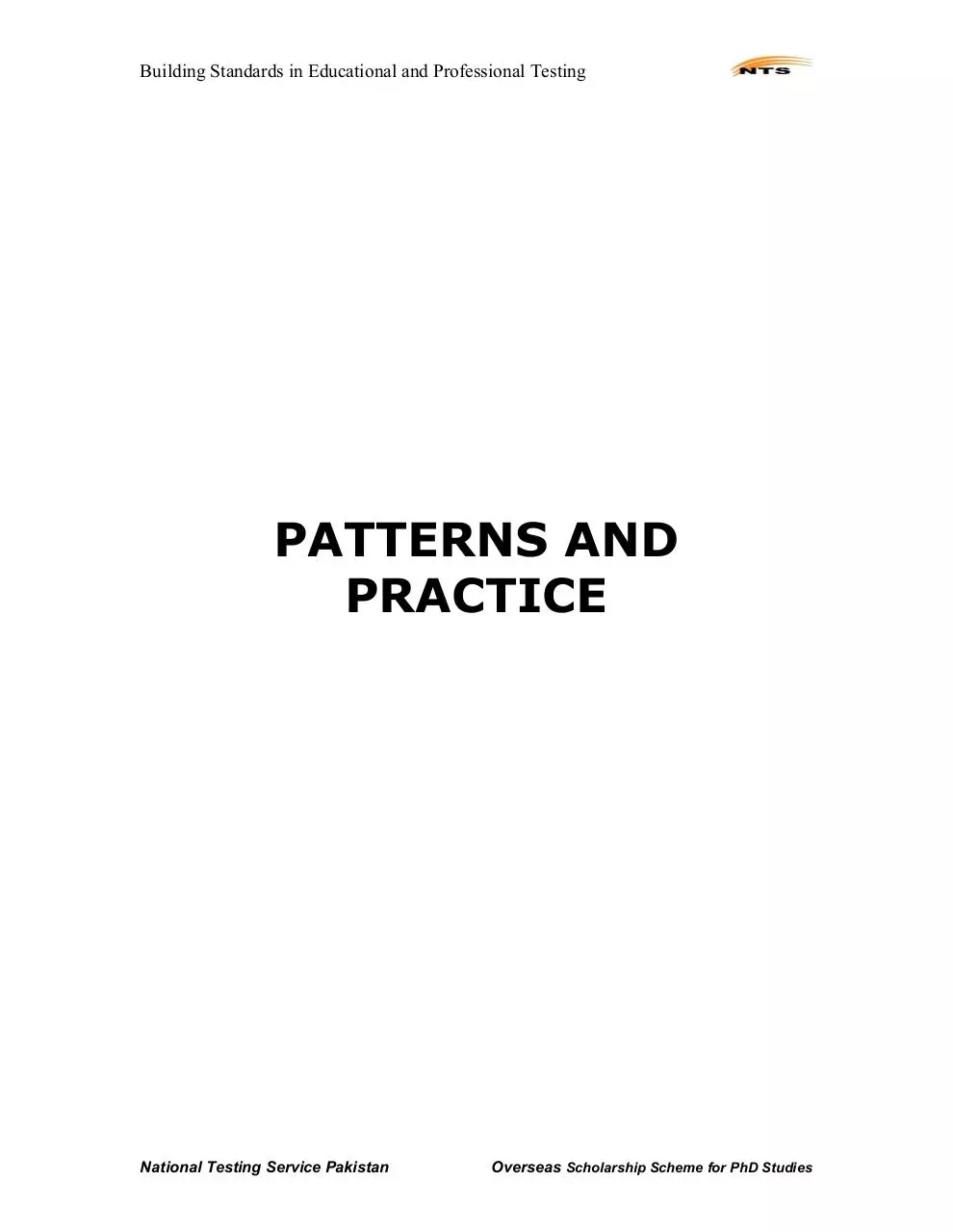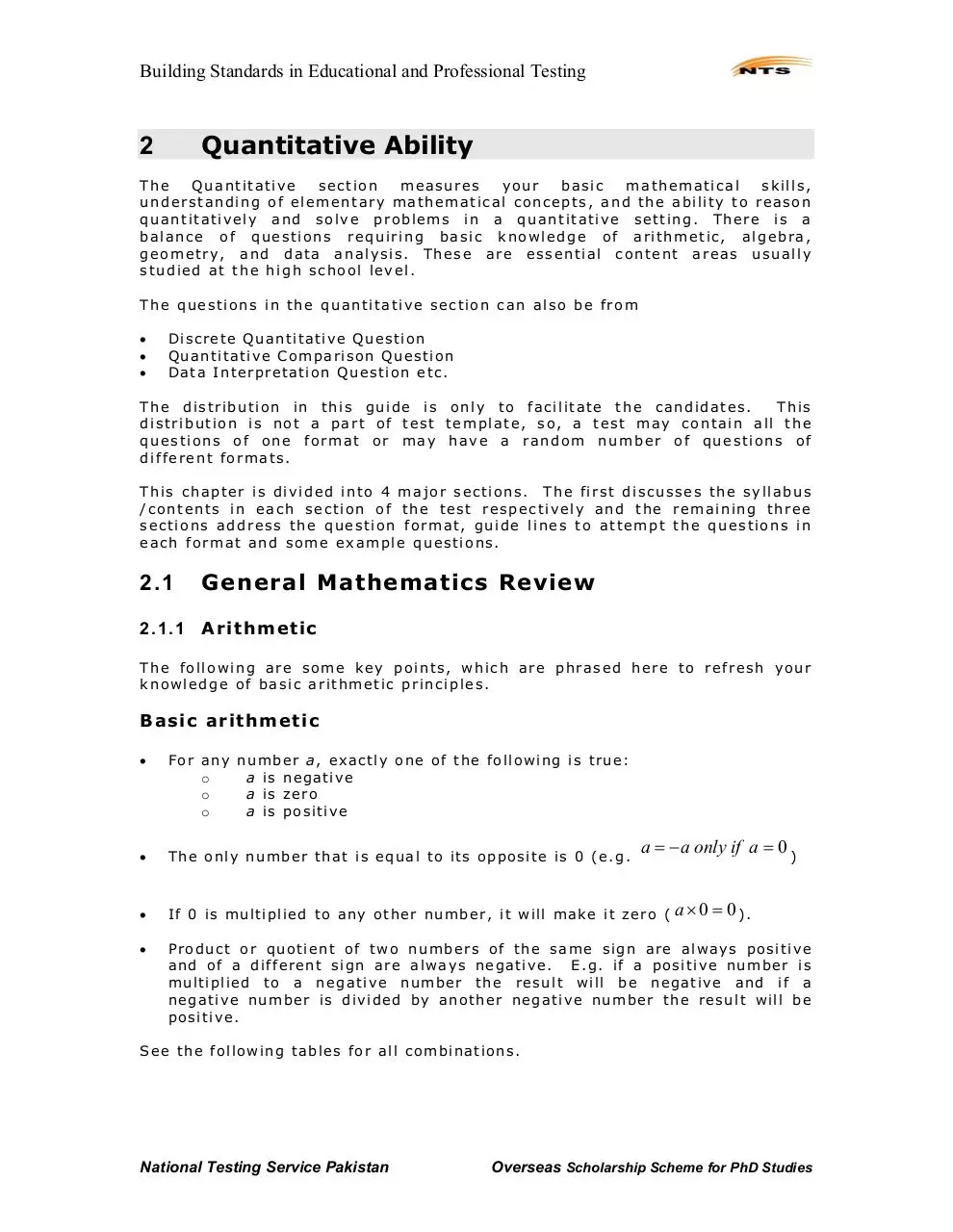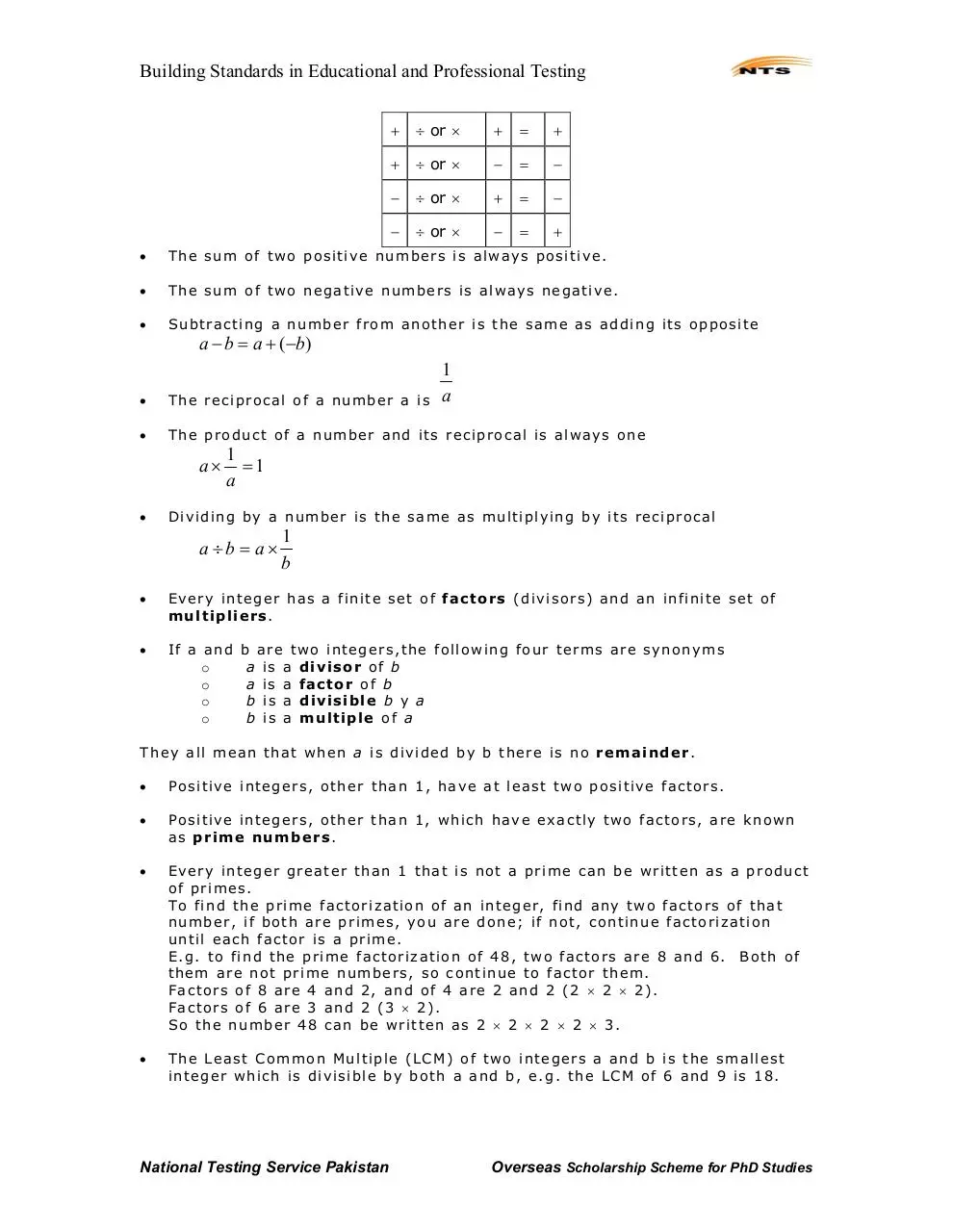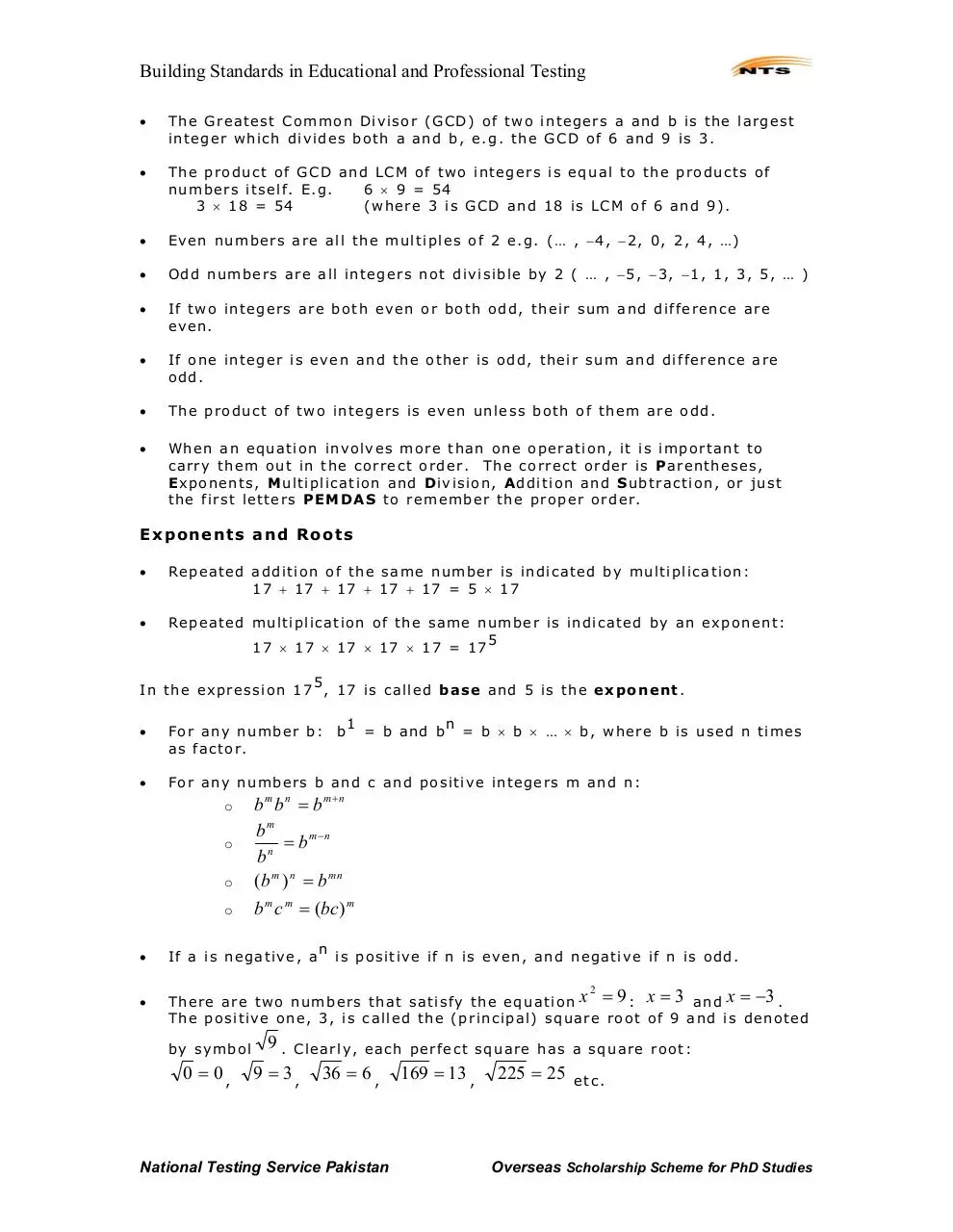# NTS Book for GAT General .pdf

### File information

Title: Microsoft Word - OVERSEAS SAMPLE PAPER & GUIDE.doc

This PDF 1.3 document has been generated by PScript5.dll Version 5.2.2 / Acrobat Distiller 5.0 (Windows), and has been sent on pdf-archive.com on 17/03/2017 at 09:14, from IP address 119.155.x.x. The current document download page has been viewed 697 times.
File size: 995.47 KB (142 pages).
Privacy: public file

### Document preview

Building Standards in Educational and Professional Testing

National Testing Service Pakistan
(Building Standards in Educational &amp; Professional Testing)

Email: correspondence@nts.org.pk

National Testing Service Pakistan

Overseas Scholarship Scheme for PhD Studies

Building Standards in Educational and Professional Testing

PATTERNS AND
PRACTICE

National Testing Service Pakistan

Overseas Scholarship Scheme for PhD Studies

Building Standards in Educational and Professional Testing

Quantitative Ability

2

The
Quantitative
section
measures
your
basic
mathematical
skills,
understanding of elementary mathematical concepts, and the ability to reason
quantitatively and solve problems in a quantitative setting. There is a
balance of questions requiring basic knowledge of arithmetic, algebra,
geometry, and data analysis. These are essential content areas usually
studied at the high school level.
The questions in the quantitative section can also be from

Di screte Quanti tative Questi on
Quanti tative Compari son Questi on
Data Interpretati on Questi on etc.

The distribution in this guide is only to facilitate the candidates.
This
distribution is not a part of test template, so, a test may contain all the
questions of one format or may have a random number of questions of
different formats.
This chapter is divided into 4 major sections. The first discusses the syllabus
/contents in each section of the test respectively and the remaining three
sections address the question format, guide lines to attempt the questions in
each format and some example questions.

2.1

General Mathematics Review

2.1.1

Arithmetic

The following are some key points, which are phrased here to refresh your
knowledge of basic arithmetic principles.

Basic arithmetic

For any number a, exactl y one of the fol l owi ng i s true:
o
a i s n egativ e
o
a i s z ero
o
a i s posi ti ve

Th e on l y nu m ber th at i s equ al to i ts opposi te i s 0 (e. g.

If 0 i s m ul ti pli ed to any oth er n um ber, i t will m ak e i t zero ( a × 0 = 0 ) .

a = − a only if a = 0 )

Product or quoti ent of two numbers of t h e same si gn are al ways posi ti ve
and of a d i fferent si gn are al ways negati ve. E. g. i f a po si ti ve number i s
mul ti pli ed to a negati ve number the r esul t wi ll be nega ti ve and i f a
negati ve number i s d i vi ded by another ne gati ve number the r esul t will be
posi ti ve.

S ee th e f oll owi n g tables f or all com bi n ati ons.

National Testing Service Pakistan

Overseas Scholarship Scheme for PhD Studies

Building Standards in Educational and Professional Testing
+

÷ or ×

+

=

+

+

÷ or ×

=

÷ or ×

+

=

÷ or ×

=

+

The sum of two posi tive numbers i s al ways posi ti ve.

The sum of two negative numbers i s al ways negati ve.

S u btracti ng a number from another i s the same as ad di ng i ts opposi te

a − b = a + (−b)

1
a

The reci pro cal of a number a i s

The produc t of a number and i ts reci procal i s al ways one

1
=1
a

D iv i di n g by a n u m ber i s th e same as m u l ti pl yi n g by i ts reci procal

a ÷b = a×

1
b

Every i n teger has a fini te set of factor s (di v i sors) an d an i nfi ni te set of
m u l t i p l i e r s.

If a and
o
o
o
o

b are two i n tegers, the f oll owi n g four terms are synonyms
a i s a divisor o f b
a i s a factor o f b
b i s a divisible b y a
b i s a multiple o f a

They al l mean that when a i s divi ded by b there i s no r e m a i n der .

Posi ti ve i n tegers, other than 1, have at l east two posi tive factors.

Posi ti ve i n tegers, other than 1, whi ch have exactl y two factors, are known
as p r i m e nu m b e r s.

Every i n teger greater than 1 that i s not a pri m e can be wri tten as a product
of pri m es.
T o f i n d t h e p r i m e f a c to r i z a ti o n of a n i n t e g e r , fi n d a n y t w o f a c t o r s o f t h a t
number, i f both are pr i m es, you are done; i f not, continue factorizati on
until each factor i s a pri m e.
E. g. to fi nd the pri m e factori z ati on of 48, two factors are 8 and 6. Both of
them are not pri m e numbers, so conti nue to factor them.
Factors of 8 are 4 and 2, and of 4 are 2 and 2 (2 × 2 × 2).
Factors of 6 are 3 and 2 (3 × 2).
S o the number 48 ca n be wri tten as 2 × 2 × 2 × 2 × 3.

Th e Leas t C om m on Mu l ti pl e (LCM) of tw o in tegers a a n d b i s th e sm all est
i n teger which i s di vi sibl e by both a and b, e. g. the LCM of 6 and 9 i s 18.

National Testing Service Pakistan

Overseas Scholarship Scheme for PhD Studies

Building Standards in Educational and Professional Testing

The Greatest Common Di vi sor (GCD) of tw o integers a and b is th e largest
i n teger which di vi des both a and b, e. g. the GCD of 6 and 9 i s 3.

The produc t of GCD an d LCM of two i n tegers i s equ al to th e prod u cts of
n u m bers i tsel f . E. g.
6 × 9 = 54
3 × 18 = 54
(where 3 i s GCD and 18 i s LCM of 6 and 9).

Even numbers are al l the mul ti ples of 2 e. g. (… , −4, −2, 0, 2, 4, …)

Odd numbe rs are all integers no t di vi si bl e by 2 ( … , −5, −3, −1, 1, 3, 5, … )

If two i n tegers are bo th even or both odd, t h ei r sum and di fference are
even.

If one i n teger i s even and the other i s odd, thei r sum and di fference are
odd.

The produc t of two i n tegers i s even unl ess both of them are odd.

When an equati on invol v es more than one operati on, i t i s i m portant to
carry them out i n the correct order. The correct order i s P arentheses,
Exponents, Mu l ti pli cati on an d Div i si on , Add i ti on an d Subtracti on, or just
the fi rst l etters PEM D AS to remember th e proper or der.

E x p o n e n ts a nd R o o ts

Repe ated a ddi ti on of the same number i s indi cated by mul ti pli cation:
17 + 17 + 1 7 + 17 + 17 = 5 × 17

Repe ated mul ti pli cation of the same number i s i n di cated by an e x ponent:
5
17 × 17 × 17 × 17 × 17 = 17

5
In the expressi on 17 , 17 i s called base and 5 i s the e x p o n e n t.

For any numbers b an d c and po si tive integers m and n:

o
o
o

= b and b

n

For any number b:
as factor.

o

b

1

= b × b × … × b, where b i s used n ti mes

b m b n = b m+n
bm
= b m−n
n
b
(b m ) n = b mn
b m c m = (bc) m
n

If a is negative, a

i s posi ti v e i f n i s ev en , an d n egati v e i f n i s odd.

There are t w o numbers that sati sf y the equati on x = 9 : x = 3 a n d x = −3 .
The posi ti ve one, 3, is call ed the (pri nci pal ) square root of 9 and i s denoted
2

by symbol

9.

0 = 0,

9 = 3,

Cl earl y, each perfect square has a square root:

36 = 6 ,

National Testing Service Pakistan

169 = 13 ,

225 = 25

etc.

Overseas Scholarship Scheme for PhD Studies

Building Standards in Educational and Professional Testing

For any posi tive number a there i s a posi tive number b that sati sfi es the
equati on

a =b.

( a )2 = a × a = a .

For any positive integer,

For any posi tive numbers a and b:

ab = a × b

a
=
b

and

o

a
b

a+b ≠ a + b
o

as 5 = 25 = 9 + 16 ≠ 9 + 16 = 3 + 4 = 7
( a )2 = a ,

Al th o u g h i t i s al w a y s t r u e t h a t
posi ti ve as

n

F o r a n y n um b e r a ,

For any number a, b and c:

an = a 2 .

a(b + c) = ab + ac
and i f

a(b − c) = ab − ac

a≠0

(b + c) b c
= +
a
a a

true only if a i s

(−5) 2 = 25 = 5 ≠ −5

a2 = a is

(b − c) b c
= −
a
a a

I ne qua li ti e s

For any number a and b, exactl y one of the foll owi n g i s true:

a&gt;b

a=b

or

or

a &lt; b.

F o r a n y n um b e r a a n d b ,

a &gt; b means

that

a − b is

positi v e.

F o r a n y n um b e r a a n d b ,

a &lt; b means

that

a − b is

n egati v e.

F o r a n y n um b e r a a n d b ,

a = b means

that

a − b is

z ero.

The symbol

means greater than or equal to and the symbol

than or equal to. E.g. the statement
number gr eater than 5.
The statem ent

2 &lt; x &lt; 5 is

x ≥ 5 means

an abbrev i ati on of

2 &lt; x and x &lt; 5 .

A ddi ng or subtracti n g a number to an i n equali ty preserves i t.

If

t h en

means l ess

that x can be 5 or any

a&lt;b,

a + c &lt; b + c and a − c &lt; b − c .

National Testing Service Pakistan

Overseas Scholarship Scheme for PhD Studies

Building Standards in Educational and Professional Testing
e. g.

a &lt; b and

c&lt;d,

then

a+c &lt;b+d .

Mul ti pl yi n g or di vi di ng an i n equali ty by a posi ti ve number pres e rves i t. If

a &lt; b and c i s

5 − 10 &lt; 6 − 10

and

A ddi n g i n equ al i ti es i n sam e di recti on preserv es i t:
If

5 &lt; 6 ⇒ 5 + 10 &lt; 6 + 10

a posi tive number, then

a×c &lt; b×c

a b
&lt;
c c.

and

Mul ti pl yi n g or di vi di ng an i n equali ty by a negati ve number reve rses i t. If

a &lt; b and c i s

a negati ve number, then

a×c &gt; b×c

and

a b
&gt;
c c.

If si des of an i n equality are both posi ti ve and both ne gati ve, taki ng the
reci procal reverses th e i n equali ty .

If

0 &lt; x &lt; 1 and a i s

If

0 &lt; x &lt; 1 and m and n are

If

0 &lt; x &lt; 1,

If

t h en

0 &lt; x &lt; 1 , then

pos i t i v e , t h en

xa &lt; a .

integers wi th

m&gt;n,

t h en

xm &lt; xn &lt; x .

x &gt; x.

1
1
&gt;x
&gt;1
x
and x

Pro per ties of Ze ro

0 i s the only number that i s nei ther negati ve nor posi ti ve.

0 i s smal l er than every posi tive number and great er than every negati ve
number.

0 i s an even i n teger.

0 i s a m ul ti pl e of ev ery i n teger.

For every number

a

:

For every number

a

: a×0 = 0.

For every posi ti ve i n teger

For every number

a

(incl u di ng 0):

For every number

a

(other than 0):

a + 0 = a and a − 0 = a .

National Testing Service Pakistan

n : 0n = 0 .

a ÷ 0 and

0÷a =

a
0

are undefi n ed symbol s.

0
= 0.
a

Overseas Scholarship Scheme for PhD Studies

Building Standards in Educational and Professional Testing

0 i s th e only n u m ber th at i s equ al to i ts opposi te:

I f t h e p r o d u c t o f t w o o r m o r e n u m b e r s i s 0 , a t l e a s t on e o f t h e m i s 0 .

0 = −0

.

Properties of One

a
a : a ×1 = a a n d = a .
1
n
n
1
=
1
:
.
number

For any number

For any

1 i s the di vi sor of every i n teger.

1 i s the small est posi ti ve i n teger.

1 i s an odd i n teger.

1 i s n ot a pri m e.

Fractions and Decimals

Wh en a w h ol e i s di vi ded i n to n equ al parts, each part i s cal l ed on e n th of
the whol e, wri tten

1
.
n

For ex am pl e, i f a pi z z a i s cu t (di vi ded) i n to 8 equ al

sli ces, each sli ce i s one ei ghth (

1
)
8

of the pi zza; a day is di vi ded i n to 24

eq ua l h o u r s , s o a n h ou r i s one tw e nt y -fourt h

1
) of
12
8
)
twenty-fourth (
24

one twel fth (

1
)
24

of a day and an inch i s

a f o ot. I f one work s for 8 hours a d a y , he works e ig ht
of a day . If a hock ey sti ck i s 40 i n ches l on g, i t

(

m e a s u r e s f o r t y t w e l f th s

(

The numbe rs such as

1
,
8

40
) of a
12
1
8
,
24 24

f o ot.
and

40
,
12

in w hi ch one i n teger is w ri tten

over the second i n teger, are called fr actio n s . The center li ne i s call ed the
fracti on bar. The number abov e the bar i s call ed the n u m e r a to r , a n d t he
number bel ow the bar i s call ed d e n o m i n a t o r .

The denom i nator of a fracti on can never be 0.

A f r a c ti o n , s u c h a s

1
,
24

in whi ch the denomi nator i s greater than

n u m e r a t o r , i s k n o w n a s a proper fraction . Its val u e is l ess than one.

A f r a c ti o n , s u c h a s

40
,
12

i n whi ch t he d e nomi na t or i s l ess t ha n nume ra t or, i s

k n o w n a s an improper fraction . Its val u e is greater t h an one.

National Testing Service Pakistan

Overseas Scholarship Scheme for PhD Studies

Building Standards in Educational and Professional Testing
12
12

A f r a c ti o n , s u c h a s ,

in whi ch the denomi nator i s equal to the nu merator,

Every fracti on can be express ed i n deci mal form (or as a whol e number) by
di vi di ng the number by the denomi nator.

i s al s o k n ow n a s a n im proper fraction . B u t, Its v al ue i s on e.

3
= 0.3,
10

3
8
48
100
= 0.75,
= 1,
= 3,
= 12.5
4
8
16
8

Unli ke the exampl es above, when most fracti ons are converted to deci mal s,
the di vi si on does not termi n ate, after 2 or 3 or 4 deci mal pl aces; rather i t
goes on forever wi th some set of di gi ts repeati n g i t.

2
3
5
17
= 0.66666...,
= 0.272727...,
= 0.416666...,
= 1.133333...
3
11
12
15

National Testing Service Pakistan

Overseas Scholarship Scheme for PhD StudiesNTS Book for GAT General.pdf (PDF, 995.47 KB)

### Share on social networks

#### HTML Code

Copy the following HTML code to share your document on a Website or Blog